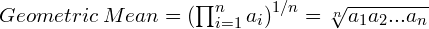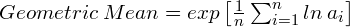## A Close Look at the Math Behind Leveraged ETF Returns

Apr 292011

#### An Application of the Geometric and Arithmetic Means

Over the past several years there has been a proliferation of ETFs for every imaginable purpose.

One particularly interesting type of ETF is the leveraged ETF. These ETFs are designed to provide 2X or 3X the daily return of a target index such as the S&P500, and they do a reasonable job of achieving this goal.

For example, if the S&P500 is up 1% for the day, the 2X S&P500 fund will be up 2% and the 3X fund will be up 3%. If the S&P500 is down 0.5% for the day, then the 2X fund will be down 1% and the 3X fund will be down 1.5%. (Note that for simplicity I’m not considering the fees and implicit borrowing costs associated with leveraged funds in this post).

Based on this description, you might think that if the S&P500 is up 10% for the year, then the 2X S&P500 fund would be up 20% and the 3X S&P500 fund will be up 30%. However, this is not the case. In fact, under some conditions, the 2X and 3X leveraged funds may be up even less than the S&P500 index. How can this be? If the daily leveraged returns are 2X or 3X the returns of the target fund, then why won’t the annual returns be 2X or 3X the annual returns?

To understand why there is discrepancy between daily returns and long-term returns, we need to review the difference between the arithmetic and geometric means.

##### Arithmetic Mean

The arithmetic mean is what most of us think of when we hear the term “average”, and it is defined by this equation.##### Geometric Mean

The geometric mean is the nth root of the product of n numbers, and it is defined by this equation:The geometric mean can also be calculated using logarithms:The geometric mean only applies for positive numbers. So, when using investment returns, the geometric mean is calculated using the decimal multiplier equivalent values. For example, a 6% return is 1.06, and a -4% return is 0.96.